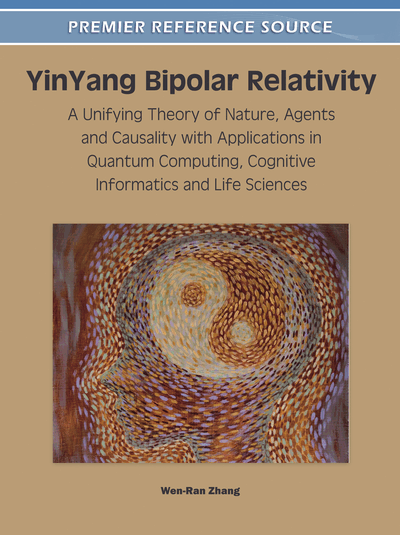# Bipolar Sets and YinYang Bipolar Dynamic Logic (BDL)

DOI: 10.4018/978-1-60960-525-4.ch003
Available
\$37.50
No Current Special Offers

## Abstract

In this chapter an equilibrium-based set-theoretic approach to mathematical abstraction and axiomatization is presented for resolving the LAFIP paradox (Ch. 1) and for enabling logically definable causality (Ch. 2). Bipolar set theory is formally presented, which leads to YinYang bipolar dynamic logic (BDL). BDL in zeroth-order, 1st-order, and modal forms are presented with four pairs of dynamic De Morgan’s laws and a bipolar universal modus ponens (BUMP). BUMP as a key element of BDL enables logically definable causality and quantum computing. Soundness and completeness of a bipolar axiomatization are asserted; computability is proved; computational complexity is analyzed. BDL can be considered a non-linear bipolar dynamic generalization of Boolean logic plus quantum entanglement. Despite its non-linear bipolar dynamic quantum property, it does not compromise the basic law of excluded middle. The recovery of BDL to Boolean logic is axiomatically proved through depolarization and the computability of BDL is proved. A redress on the ancient paradox of the liar is presented with a few observations on Gödel’s incompleteness theorem. Based on BDL, bipolar relations, bipolar transitivity, and equilibrium relations are introduced. It is shown that a bipolar equilibrium relation can be a non-linear bipolar fusion of many equivalence relations. Thus, BDL provides a logical basis for YinYang bipolar relativity–an equilibrium-based axiomatization of social and physical sciences.
Chapter Preview
Top

## Introduction

In mathematics, a set is any collection of objects (elements) considered as a whole. Although this appears to be a simple idea, set is actually one of the most fundamental concepts in modern logical systems and mathematics that forms the foundation of information and computation.

The principle of mathematical abstraction in classical set theory was rarely challenged throughout history. The principle claims that the concept of an element is self-evident without the need for proof of any kind and the properties of a set are independent of the nature of its elements. Classical logic is based on this principle. Thus, a classical (crisp) set X in a universe U can be defined in the form of its characterization function μX:U→{0,1} which yields the value “1” or “true” for the elements belonging to the set X and “0” or “false” for the elements excluded from the set X. Evidently, classical set theory is based on Aristotle’s universe of truth objects as defined in the unipolar bivalent space {0,1}. Since the principle has been rarely questioned in history it is commonly considered “unquestionable”.

The LAFIP paradox (Zhang 2009a) (Ch. 1) presents a fundamental challenge to the principle of classical mathematical abstraction. The challenge is aimed at searching for a new mathematical abstraction that leads to logically definable causality, axiomatization of physics, axiomatization of agent interaction, and a logical foundation for quantum gravity. As discussed in early chapters the four goals are interrelated or fundamentally equivalent.

An equilibrium-based approach to mathematical abstraction and axiomatization is presented in this chapter for resolving the LAFIP paradox. YinYang bipolar set and bipolar dynamic logic (BDL) are introduced. Soundness and completeness of the bipolar axiomatization is asserted. The recovery of BDL to Boolean logic is proved. A redress on the ancient paradox of the liar is presented with a few observations on Gödel’s incompleteness theorem. Based on BDL, bipolar relations, bipolar transitivity, and equilibrium relations are introduced. BDL and bipolar equilibrium relations provide a logical basis for YinYang bipolar relativity and bipolar quantum computing.

The remaining presentations and discussions of this chapter are organized in the following sections:

• Bipolar Sets and Bipolar Dynamic Logic (BDL). This section lays out the groundwork for this chapter. The concepts of bipolar element, bipolar set, bipolar poset, and bipolar lattice are introduced.

• Laws of Equilibrium and Bipolar Universal Modus Ponens (BUMP). This section presents equilibrium-based laws including the bipolar laws of excluded middle, four pairs of bipolar dynamic DeMorgan’s laws, and the key element of BDL – bipolar universal modus ponens (BUMP).

• Bipolar Axiomatization and Computation. This section presents a bipolar axiomatization with soundness and completeness theorems. The computability of BDL is axiomatically proved. Computational complexity is analyzed.

• Bipolar Modality. This section presents a transformation of BDL to its modal form.

• Bipolar Relations and Equilibrium Relations. This section presents the concepts of bipolar relation, bipolar transitivity, and equilibrium relation.

• On Gödel’s Incompleteness Theorem. This section gives a redress on the liar’s case “This sentence is not true” and makes a few observations on Gödel’s incompleteness theorem.

• Research Topics. This section lists a few research topics.

• Summary. This section draws a few conclusions.

## Complete Chapter List

Search this Book:
Reset